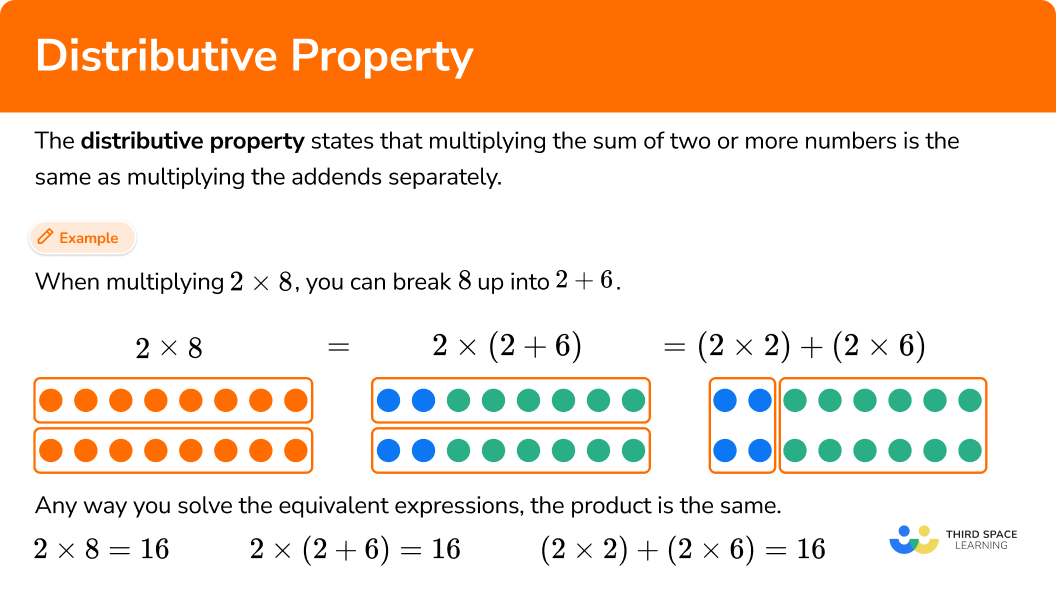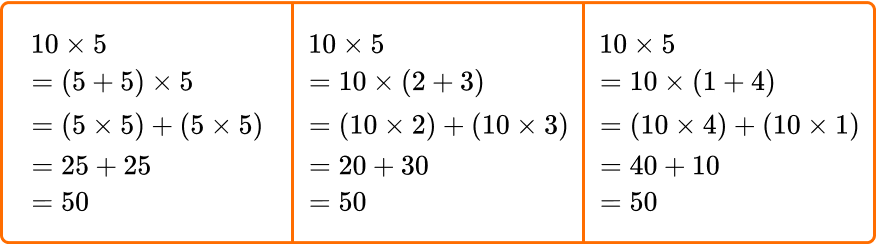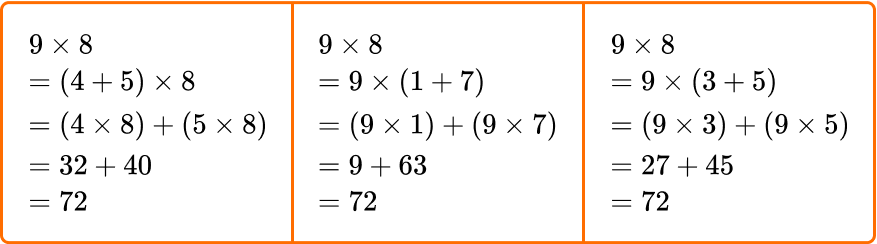# Distributive property

Here you will learn about the distributive property, including what it is, and how to use it to solve problems.

Students will first learn about the distributive property as part of operations and algebraic thinking in 3rd grade.

## What is the distributive property?

The distributive property states that multiplying the sum of two or more numbers is the same as multiplying the addends separately.

For example,

When multiplying 2 \times 8, you can break 8 up into 2 + 6.

The distributive property says that you can multiply the parts separately and then add the products together.

Any way you solve the equivalent expressions, the product is the same.

For most expressions, there is more than one way to use the distributive property.

For example,

When multiplying 2 \times 8, you can break 8 up into 5 + 3.

The distributive property says that you can multiply the parts separately and then add the products together.

Any way you solve the equivalent expressions, the product is the same.

### What is the distributive property?## Common Core State Standards

How does this relate to 3rd grade math?

• Grade 3 – Operations and Algebraic Thinking (3.OA.B.5)
Apply properties of operations as strategies to multiply and divide.
Examples: If 6 \times 4 = 24 is known, then 4 \times 6 = 24 is also known. (Commutative property of multiplication.)
3 \times 5 \times 2 can be found by 3 \times 5 = 15, then 15 \times 2 = 30, or by 5 \times 2 = 10, then 3 \times 10 = 30. (Associative property of multiplication.)
Knowing that 8 \times 5 = 40 and 8 \times 2 = 16, one can find 8 \times 7 as 8 \times (5 + 2) = (8 \times 5) + (8 \times 2) = 40 + 16 = 56. (Distributive property.)

## How to use the distributive property

In order to use the distributive property:

1. Identify an equation multiplying two numbers.
2. Show one of the numbers being multiplied as a sum of numbers.
3. Multiply each number in the sum.
4. Add the partial products together to find the final product.

## Distributive property examples

### Example 1: distributive property with basic facts

Show how to solve 3 \times 5 using the distributive property.

1. Identify an equation multiplying two numbers.

You can use the distributive property with 3 \times 5, since it is multiplication.

2Show one of the numbers being multiplied as a sum of numbers.

Either number can be used, but for this example let’s break up 5 into 4 + 1.

3 \times 5=3 \times(4+1)

3Multiply each number in the sum.

\begin{aligned} & 3 \times(4+1) \\\\ & =(3 \times 4)+(3 \times 1) \\\\ & =12+3 \end{aligned}

4Add the partial products together to find the final product.

12 + 3 = 15

3 \times 5=15 can be solved using the distributive property.

### Example 2: distributive property with basic facts

Show how to solve 12 \times 9 using the distributive property.

Identify an equation multiplying two numbers.

Show one of the numbers being multiplied as a sum of numbers.

Multiply each number in the sum.

Add the partial products together to find the final product.

### Example 3: distributive property with basic facts

Show how to solve 7 \times 6 using the distributive property.

Identify an equation multiplying two numbers.

Show one of the numbers being multiplied as a sum of numbers.

Multiply each number in the sum.

Add the partial products together to find the final product.

### Example 4: distributive property with basic facts

Show how to solve 4 \times 11 using the distributive property.

Identify an equation multiplying two numbers.

Show one of the numbers being multiplied as a sum of numbers.

Multiply each number in the sum.

Add the partial products together to find the final product.

### Example 5: distributive property with basic facts

Show how to solve 8 \times 5 using the distributive property.

Identify an equation multiplying two numbers.

Show one of the numbers being multiplied as a sum of numbers.

Multiply each number in the sum.

Add the partial products together to find the final product.

### Example 6: distributive property with basic facts

Show how to solve 3 \times 12 using the distributive property.

Identify an equation multiplying two numbers.

Show one of the numbers being multiplied as a sum of numbers.

Multiply each number in the sum.

Add the partial products together to find the final product.

### Teaching tips for the distributive property

• Intentionally choose practice problems that lend themselves to being solved with the distributive property, as it is not always necessary or useful in all solving situations.

• Instead of just giving students the distributive property definition, draw attention to examples of the distributive property as they come up in daily math activities. You may even keep an anchor chart of different examples. Over time, students will start using it and recognizing it on their own and then you can introduce them to the property and its official definition through their own examples.

• Include plenty of student discourse around this topic to ensure that students understand that breaking apart a number and then multiplying it in parts does not change the total product. This could include students sharing their thinking or critiquing the thinking of others.

### Easy mistakes to make

• Thinking there is only one way to use the distributive property to solve
Often, there is more than one way to use the distributive property when solving.
For example,

\begin{aligned} & 4 \times 5 \hspace{4.65cm} 4 \times 5 \\ & =(2+2) \times 5 \hspace{3.5cm} =(1+3) \times 5 \\ & =(2 \times 5)+(2 \times 5) \hspace{1cm} \text{ OR } \hspace{1cm} =(1 \times 5)+(3 \times 5) \\ & =10+10 \hspace{3.9cm} =5+15 \\ & =20 \hspace{4.63cm} =20 \end{aligned}

To use the distributive property, break apart a number being multiplied with addition. Using multiplication can lead to the incorrect answer.
For example,

• Not decomposing by place value
To use the distributive property, break apart a number being multiplied in a way that keeps the overall value the same. This includes preserving place value. Otherwise the products will not be equivalent.
For example,

### Practice distributive property questions

1. Which of the following equations shows 12 \times 6 using the distributive property?

(10 \times 2) \times 6=(10 \times 2)+(10 \times 6)12 \times(2+4)=12 \times 2+2 \times 4(1 \times 2)+6=(1 \times 6)+(1 \times 2)12 \times(3+3)=(12 \times 3)+(12 \times 3)The numbers are being multiplied, so the distributive property can be used.

\begin{aligned} & 12 \times 6 \\\\ & =12 \times(3+3) \hspace{1.2cm} \text{ *Break 6 up into 3 + 3}\\\\ & =(12 \times 3)+(12 \times 3) \hspace{0.3cm} \text{ *Multiply each 3 by 12} \\\\ & =36+36 \hspace{1.8cm} \text{ *Add the partial products back together} \\\\ & =72 \end{aligned}

2. Which of the following equations shows 7 \times 9 using the distributive property?

(1+7) \times 9=(1 \times 9)+(7 \times 9)7 \times(3 \times 3)=(7 \times 3)+(7 \times 3)(4+3) \times 9=(4 \times 9)+(3 \times 9)7 \times(6+3)=7 \times 6+6 \times 3The numbers are being multiplied, so the distributive property can be used.

\begin{aligned} & 7 \times 9 \\\\ & =(4+3) \times 9 \hspace{1.2cm} \text{ *Break 7 up into 4 + 3}\\\\ & =(4 \times 9)+(3 \times 9) \hspace{0.4cm} \text{ *Multiply both 4 and 3 by 9} \\\\ & =36+27 \hspace{1.6cm} \text{ *Add the partial products back together} \\\\ & =63 \end{aligned}

3. Which of the following equations shows 11 \times 8 using the distributive property?

(1+10) \times 8=(1 \times 8)+(10 \times 8)(1+1) \times 8=(1 \times 8)+(1 \times 8)11 \times(4 \times 2)=(11 \times 4)+(11 \times 2)11 \times(4+4)=(11 \times 4)+4The numbers are being multiplied, so the distributive property can be used.

\begin{aligned} & 11 \times 8 \\\\ & =(1+10) \times 8 \hspace{1.1cm} \text{ *Break 11 up into 1 + 10}\\\\ & =(1 \times 8)+(10 \times 8) \hspace{0.3cm} \text{ *Multiply both 1 and 10 by 8} \\\\ & =8+80 \hspace{1.8cm} \text{ *Add the partial products back together} \\\\ & =88 \end{aligned}

4. Which of the following equations shows 3 \times 7 using the distributive property?

(2+1) \times 7=(2 \times 7)+(2 \times 1)3 \times(2+2+3)=(3 \times 2)+(3 \times 2)3 \times(1+3+3)=(3 \times 1)+(3 \times 3)+(3 \times 3)(3 \times 1) \times 7=3 \times(1 \times 7)The numbers are being multiplied, so the distributive property can be used.

\begin{aligned} & 3 \times 7 \\\\ & =3 \times(1+3+3) \hspace{1.8cm} \text{ *Break 7 up into 1 + 3 + 3}\\\\ & =(3 \times 1)+(3 \times 3)+(3 \times 3) \hspace{0.3cm} \text{ *Multiply 1, 3 and 3 by 3} \\\\ & =3+9+9 \hspace{2.6cm} \text{ *Add the partial products back together} \\\\ & =21 \end{aligned}

5. Which of the following equations is NOT a way to solve 10 \times 5 using the distributive property?

(5+5) \times 5=(5 \times 5)+(5 \times 5)(2 \times 5) \times 5=(2 \times 5)+(2 \times 5)10 \times(2+3)=(10 \times 2)+(10 \times 3)10 \times(1+4)=(10 \times 4)+(10 \times 1)\begin{aligned} & 10 \times 5 \\\\ & =(2 \times 5) \times 5 \hspace{1.2cm} \text{ *This breaks up 10, but not into a sum} \\\\ & =(2 \times 5)+(2 \times 5) \hspace{0.4cm} \text{ *This is equal to } (2 + 2) \times 5, \text{ but not } (2 \times 5) \times 5 \end{aligned}

This strategy is NOT a way to solve with the distributive property.

All the other equations break 10 or 5 up into a sum and add the products of the parts, using the distributive property correctly:6. Which of the following equations is NOT a way to solve 9 \times 8 using the distributive property?

(4+5) \times 8=(4 \times 8)+(5 \times 8)9 \times(1+7)=(9 \times 1)+(9 \times 7)9 \times(3+5)=(9 \times 3)+(9 \times 5)(2+6) \times 8=(2 \times 8)+(6 \times 8)\begin{aligned} & 9 \times 8 \\\\ & =(2+6) \times 8 \hspace{1.2cm} \text{ *This breaks up 8 instead of 9} \\\\ & =(2 \times 8)+(6 \times 8) \hspace{0.4cm} \text{ *This is equal to } (2 + 6) \times 8, \text{ or } 8 \times 8 \text{ not } 9 \times 8 \end{aligned}

This strategy is NOT a way to solve 9 \times 8 with the distributive property.

All the other equations break 9 or 8 up into a sum and add the products of the parts, using the distributive property correctly:## Distributive property FAQs

Does the distributive property work with other groupings of numbers besides whole numbers?

Yes, the distributive property can be used with integers (including negative numbers) and rational numbers (including fractions and decimals), as long as the numbers are all being multiplied. In middle and high school, students will learn how to use the distributive property with any real number and/or algebraic expression.

Is the distributive property the same as the associative property?

No, even though the associative property also uses parentheses, they are different properties. The associative property says you can change the grouping of numbers when adding or multiplying and the sum or product will be the same. This is different from the distributive property.

Can you use the distributive property with subtraction?

Yes, this is called the distributive property of multiplication over subtraction.

What is the distributive law of multiplication?

This is a general term and means the same as the distributive property.

Do you have to use parentheses when writing equations for the distributive property?

No, because of the order of operations (or PEMDAS), the products will be found first and then added together. However, it is good practice to group each partial product with parentheses.

## Still stuck?

At Third Space Learning, we specialize in helping teachers and school leaders to provide personalized math support for more of their students through high-quality, online one-on-one math tutoring delivered by subject experts.

Each week, our tutors support thousands of students who are at risk of not meeting their grade-level expectations, and help accelerate their progress and boost their confidence.